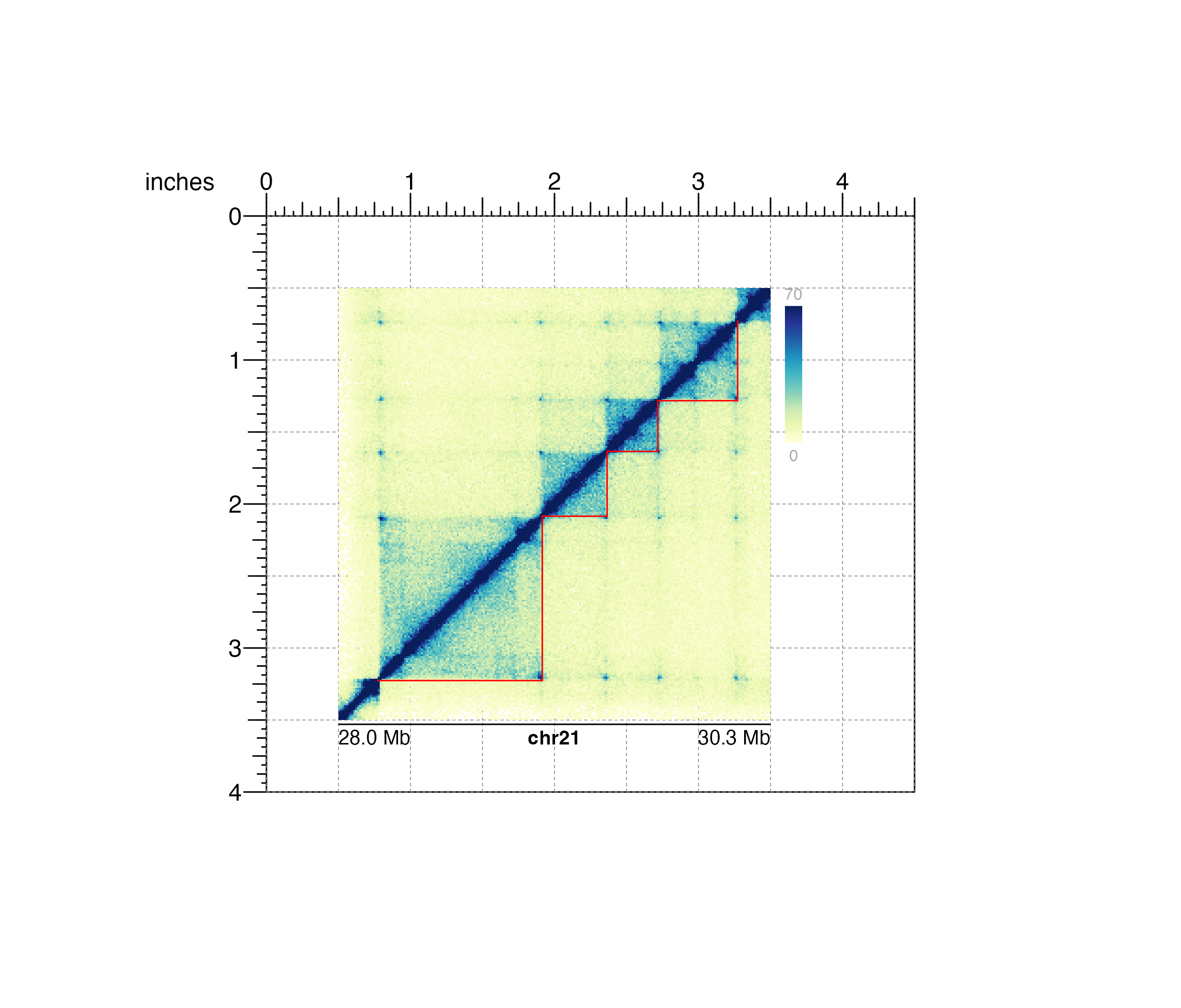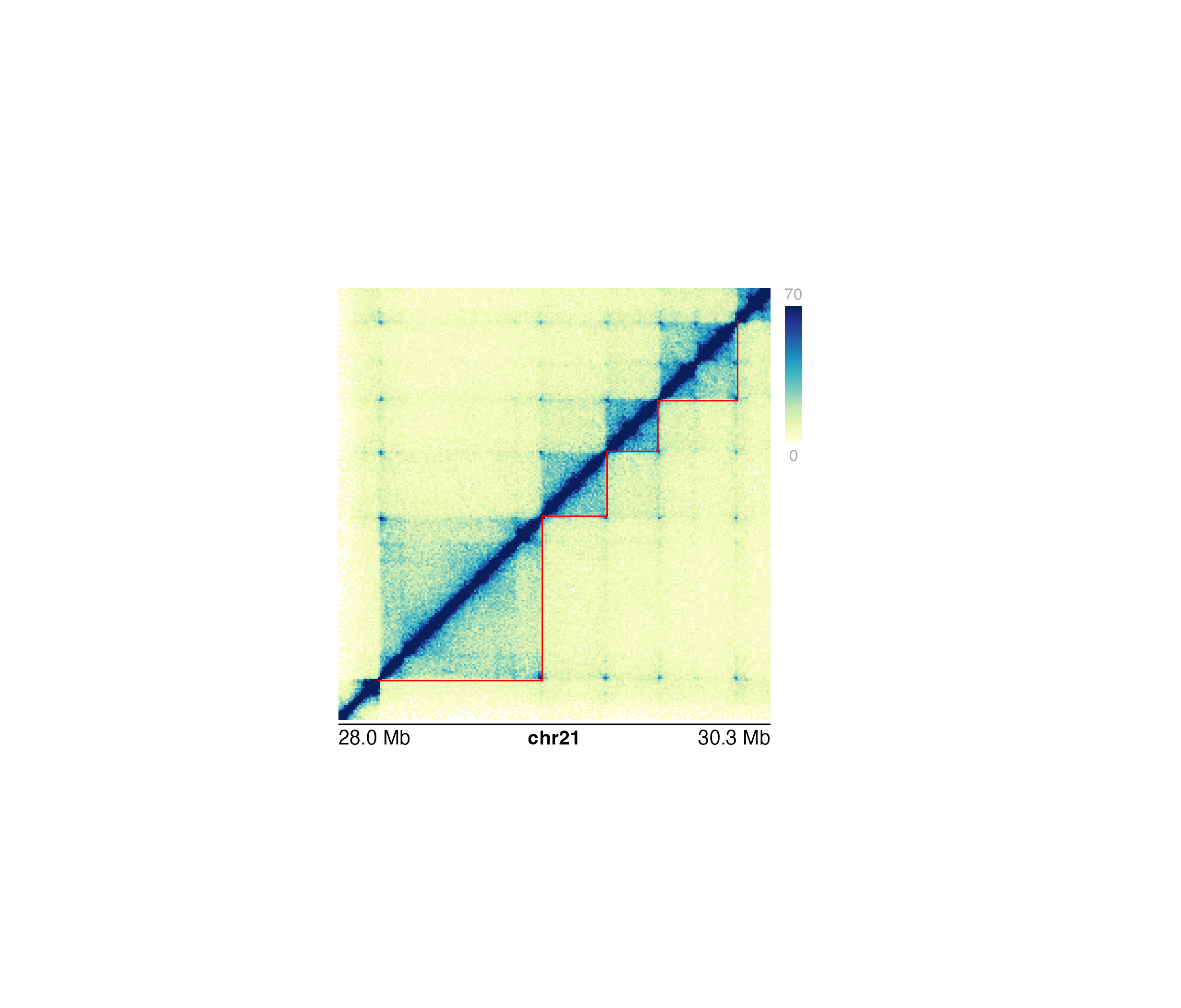Annotate domains in a Hi-C plot

annoDomains(
plot,
data,
half = "inherit",
linecolor = "black",
params = NULL,
...
)

## Arguments

plot

Hi-C plot object from plotHicSquare or plotHicTriangle on which to annotate pixels.

data

A string specifying the BED file path, a dataframe in BED format, or a GRanges object specifying domain ranges.

half

Character value specifying which half of hic plots to annotate. Triangle Hi-C plots will always default to the entirety of the triangular plot. Default value is half = "inherit". Options are:

• "inherit": Domains will be annotated on the half inherited by the input Hi-C plot.

• "both": Domains will be annotated on both halves of the diagonal of a square Hi-C plot.

• "top": Domains will be annotated on the upper diagonal half of a square Hi-C plot.

• "bottom": Domains will be annotated ont the bottom diagonal half of a square Hi-C plot.

linecolor

A character value specifying the color of the domain annotations. Default value is linecolor = "black".

params

An optional pgParams object containing relevant function parameters.

...

Additional grid graphical parameters. See gpar.

## Value

Returns a domain object containing relevant genomic region, placement, and grob information.

## Examples

## Define a GRanges object with TAD ranges
library(GenomicRanges)
library(IRanges)
domains <- GRanges("chr21",
ranges = IRanges(
start = c(28210000, 29085000, 29430000, 29700000),
end = c(29085000, 29430000, 29700000, 30125000)
)
)

library(plotgardenerData)
data("IMR90_HiC_10kb")

## Create page
pageCreate(width = 4.5, height = 4, default.units = "inches")

## Plot and place a square Hi-C plot
hicPlot <- plotHicSquare(
data = IMR90_HiC_10kb, resolution = 10000,
zrange = c(0, 70),
chrom = "chr21",
chromstart = 28000000, chromend = 30300000,
assembly = "hg19",
x = 0.5, y = 0.5, width = 3, height = 3,
just = c("left", "top"),
default.units = "inches"
)
#> Read in dataframe.  Assuming 'chrom' in column1 and 'altchrom' in column2. 10000 BP resolution detected.
#> hicSquare[hicSquare1]

## Annotate domains on bottom half 0f Hi-C plot
annoDomains(
plot = hicPlot, data = domains,
half = "bottom", linecolor = "red"
)
#> domain[domain1]

## Annotate heatmap legend
annoHeatmapLegend(
plot = hicPlot,
x = 3.6, y = 0.5, width = 0.12, height = 1.2,
just = c("left", "top"), default.units = "inches"
)
#> heatmapLegend[heatmapLegend1]

## Annotate genome label
annoGenomeLabel(
plot = hicPlot, x = 0.5, y = 3.53, scale = "Mb",
just = c("left", "top")
)
#> genomeLabel[genomeLabel1]## Hide page guides
pageGuideHide()